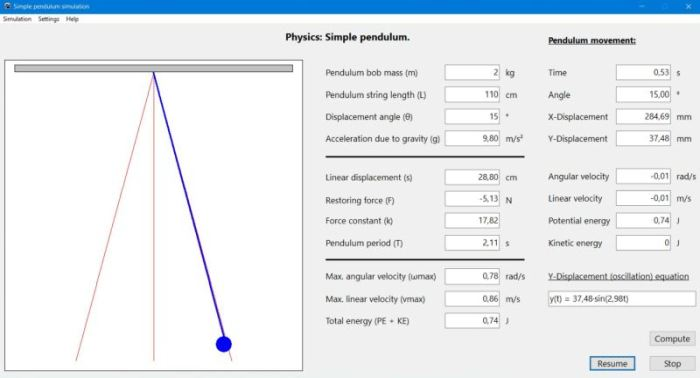# Computing: Free Pascal Programming

 Programming Home Home Contact

## Simulation of a simple pendulum (Pendulum).

Description: Physics application, dealing with simple pendulums. For a pendulum of known mass, length and displacement angle, the application calculates all pendulum relevant values, including restore force and energy. The movement of the pendulum may then be simulated, in real time or slow motion, with not only its xy-position, but also the velocity varying as in reality (functions of time). The values of the pendulum's potential and kinetics energy are updated at each simulation step, too.
Beside the possibility to manually pause the simulation, the pendulum may automatically be holded, when it passes through its lowest position (y=0; angle=0) and its two heighest positions (y=ymax; angle=displacement angle). This gives the user the possibility to study the values at these particular points (cf. the application help text for further information).

Issues: Detection of the lowest pendulum position is actually mostly missed and the pendulum only pauses its movement randomly at this point. Running the simulation slower should help.

Free Pascal features: Drawings using bitmap canvas. Using a timer to create time-dependent drawings in order to realize simple graphical animations (simulations).

Screenshot: